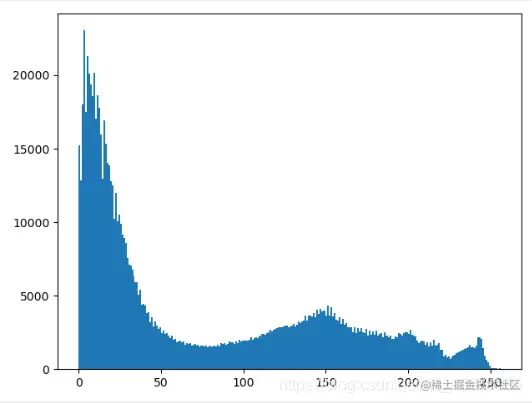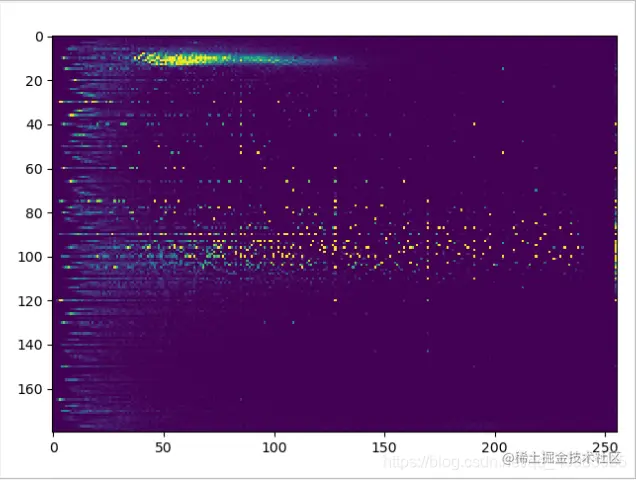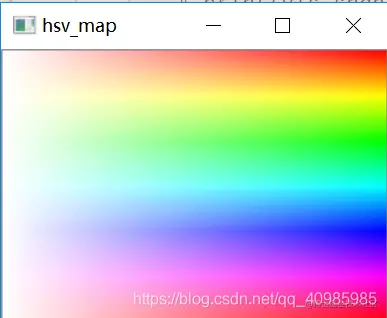# Use Python and OpenCV to calculate and draw two-dimensional histogram

2022-01-30 17:12:46

# Use Python,Opencv Calculate and draw the two-dimensional histogram

This blog will show you how to use Python,Opencv Calculate and draw the two-dimensional histogram （ Use them separately Opencv and Numpy Calculation ）, Two dimensional histogram can give us a better understanding of different pixel densities .

## 1. design sketch

The original figure is as follows ：1 The dimensional histogram is as follows ：2 The dimensional histogram is as follows ： X Axis display S value ,Y Axis display tone .hsvmap The renderings are as follows ：## 2. Source code

``````# OpenCV 2-D histogram in : Use the same function cv2.calcHist（） Calculation .
#  about 1D Histogram , We from BGR Convert to grayscale
#  about 2D Histogram , You need to remove the image from BGR Convert to HSV

import cv2
import numpy as np
from matplotlib import pyplot as plt

gray = cv2.cvtColor(img,cv2.COLOR_BGR2GRAY)
hsv = cv2.cvtColor(img, cv2.COLOR_BGR2HSV)

# 1 Dimensional histogram
hist = cv2.calcHist([gray], , None, , [0, 256])
hist, bins = np.histogram(img.ravel(), 256, [0, 256])
plt.hist(img.ravel(), 256, [0, 256])
plt.show()

#  Two dimensional histogram can give us a better understanding of different pixel densities
# OpenCV Calculation 2D Histogram
# HSV Images  [0,1] Express H、S passageway ,[180,256] Express H、S Of bins Respectively 180、256
# [0,180,0,256] Represents the range of values
hist = cv2.calcHist([hsv], [0, 1], None, [180, 256], [0, 180, 0, 256])

hist = np.clip(hist * 0.005, 0, 1)
cv2.imshow('hist', hist)
cv2.waitKey(0)

plt.imshow(hist, interpolation='nearest')
plt.show()

# Numpy Calculation 1D Histogram ：np.histogram（）;
# Numpy Calculation 2D Histogram ：np.historogram2d（）
h, s, v = cv2.split(hsv)
hist, xbins, ybins = np.histogram2d(h.ravel(), s.ravel(), [180, 256], [[0, 180], [0, 256]])
plt.imshow(hist, interpolation='nearest')
plt.show()
Copy code ``````